# The PROBIT Procedure

### OUTPUT Statement

• OUTPUT <OUT=SAS-data-set keyword=name …keyword=name> ;

The OUTPUT statement creates a new SAS data set containing all variables in the input data set and, optionally, the fitted probabilities, the estimate of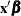, and the estimate of its standard error. Estimates of the probabilities,, and the standard errors are computed for observations with missing response values as long as the values of all the explanatory variables are nonmissing. This enables you to compute these statistics for additional settings of the explanatory variables that are of interest but for which responses are not observed.

You can specify multiple OUTPUT statements. Each OUTPUT statement creates a new data set and applies only to the preceding MODEL statement. If you want to create a SAS data set in a permanent library, you must specify a two-level name. For more information about permanent libraries and SAS data sets, see SAS Language Reference: Concepts.

Details on the specifications in the OUTPUT statement are as follows:

keyword=name

specifies the statistics to include in the output data set and assigns names to the new variables that contain the statistics. Specify a keyword for each desired statistic (see the following list of keywords), an equal sign, and the variable to contain the statistic.

The keywords allowed and the statistics they represent are as follows:

PROB | P

cumulative probability estimates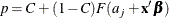STD

standard error estimates of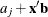XBETA

estimates of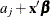OUT=SAS-data-set

names the output data set. By default, the new data set is named by using the DATAn convention.

When the single variable response syntax is used, the _LEVEL_ variable is added to the output data set, and there are k – 1 output observations for each input observation, where k is the number of response levels. There is no observation output corresponding to the highest response level. For each of the k – 1 observations, the PROB variable contains the fitted probability of obtaining a response level up to the level indicated by the _LEVEL_ variable, the XBETA variable contains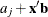, where j references the levels (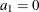), and the STD variable contains the standard error estimate of the XBETA variable. See the section Details: PROBIT Procedure for the formulas for the parameterizations.